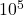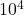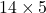How Cheenta works to ensure student success?
Explore the Back-Story

# Digits of number | PRMO 2018 | Question 3Try this beautiful problem from the PRMO, 2018 based on Digits of number.

## Digits of number - PRMO 2018

Consider all 6-digit numbers of the form abccba where b is odd. Determine the number of all such 6-digit numbers that are divisible by 7.

• is 107
• is 70
• is 840
• cannot be determined from the given information

### Key Concepts

Algebra

Numbers

Multiples

PRMO, 2018, Question 3

Higher Algebra by Hall and Knight

## Try with Hints

abccba (b is odd)

=a(+1)+b(+10)+c(+)

=a(1001-1)100+a+10b(1001)+(100)(11)c

=(7.11.13.100)a-99a+10b(7.11.13)+(98+2)(11)c

=7p+(c-a) where p is an integer

Now if c-a is a multiple of 7

c-a=7,0,-7

hence number of ordered pairs of (a,c) is 14

since b is odd

number of such number==70.

## Subscribe to Cheenta at Youtube

Try this beautiful problem from the PRMO, 2018 based on Digits of number.

## Digits of number - PRMO 2018

Consider all 6-digit numbers of the form abccba where b is odd. Determine the number of all such 6-digit numbers that are divisible by 7.

• is 107
• is 70
• is 840
• cannot be determined from the given information

### Key Concepts

Algebra

Numbers

Multiples

PRMO, 2018, Question 3

Higher Algebra by Hall and Knight

## Try with Hints

abccba (b is odd)

=a(+1)+b(+10)+c(+)

=a(1001-1)100+a+10b(1001)+(100)(11)c

=(7.11.13.100)a-99a+10b(7.11.13)+(98+2)(11)c

=7p+(c-a) where p is an integer

Now if c-a is a multiple of 7

c-a=7,0,-7

hence number of ordered pairs of (a,c) is 14

since b is odd

number of such number==70.

## Subscribe to Cheenta at Youtube

This site uses Akismet to reduce spam. Learn how your comment data is processed.

### Knowledge Partner